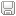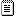Be myself :: 'crypto' 카테고리의 글 목록

•
•
•
•
•
•
• 1
• 2
• 3
• 4
• 5
• 6
• 7
• 8
• 9
• 10
• 11
• 12
• 13
• 14
• 15
• 16
• 17
• 18
• 19
• 20
• 21
• 22
• 23
• 24
• 25
• 26
• 27
• 28
• 29
• 30
•
•
•
•
•
•

1. 2017.02.05
2. 2015.11.20
3. 2015.05.07
4. 2015.04.25
5. 2015.03.21
6. 2015.02.26

[BCTF 2017]Beginner's luck (crypto 40)

crypto 2017.02.05 22:17

Recently i am interested in crypto. so I enjoyed this challenge a lot.

Look at the problem belowenc27.py

If you look at enc27.py, you can see it's xor encryption. and 24 byte block padding.png file is encrypted with key( original_png ^ key ) And as you know, A xor A = 0. so if we know original_png. then we can find key. I checked png sample files and file signature to figure out key. and enc27.py is padding 24 bit message block and xoring with key i guess key length is 24. So if I xor between png file signature and encrypted png,  it would reveals padding value..

Do it.

```import itertools

def xoring(m1,m2):
return ''.join(chr(ord(a)^ord(b)) for a,b in zip(m1,m2))

def decrypt(enc, key):
key = itertools.cycle(key)
dec = ''.join(chr(ord(a) ^ ord(b)) for a,b in zip(key,enc))
return dec

def main():
f = open("BITSCTFfullhd.png","r")
f2 = open("tmp.png","wb")

#png signature
png = "\x89\x50\x4E\x47\x0D\x0A\x1A\x0A\x00"

key = xoring(buf[:9],png)
#unkown key
key += "A"*(24-9)

dec = decrypt(buf,key)
f2.write(dec)

f.close()
f2.close()

if __name__ == '__main__':
main()

```we know 9 bytes key. And first 9 byte from each 24 byte of block was decrypted. You can see that "\x13" is repeated 4 times. Yeah~ padding value is "\x13". "\x13"*(15)^enc[-15:] would reveal left key values.

```import itertools

def xoring(m1,m2):
return ''.join(chr(ord(a)^ord(b)) for a,b in zip(m1,m2))

def decrypt(enc, key):
key = itertools.cycle(key)
dec = ''.join(chr(ord(a) ^ ord(b)) for a,b in zip(key,enc))
return dec

def main():
f = open("BITSCTFfullhd.png","r")
f2 = open("tmp.png","wb")

#png signature
png = "\x89\x50\x4E\x47\x0D\x0A\x1A\x0A\x00"

key = xoring(buf[:9],png)
key += xoring(buf[-15:], "\x13"*15)

dec = decrypt(buf,key)
f2.write(dec)

f.close()
f2.close()

if __name__ == '__main__':
main()
```flag is BITSCTF{p_en_gee}

'crypto' 카테고리의 다른 글

 [BCTF 2017]Beginner's luck (crypto 40)  (0) 2017.02.05 2015.11.20 2015.05.07 2015.04.25 2015.03.21 2015.02.26

[picoctf 2015]Repeated XOR

crypto 2015.11.20 20:26

문제는 다음과 같다.encrypted.txt를 보면 다음과 같다.hex가 잔득 적힌 txt파일이다. 파이썬으로 .decode('hex')해서 바이너리로 만든 다음에 연산하면 될 것 같다.

이 문제는 사실 알면 단순하게 바로 풀 수 있는데 워낙 크립토에 문외한인지라.. 상당히 오랜시간이 걸린 문제다. ㅠㅠ
문제 제목이 힌트인데 Repeated xor 같은 경우는 어떤 key가 주어지고, plain text의 길이만큼 concatenation되어서 문장 전체와 xor하는 암호화 기법이다. 이 것을 복호화 하는 방법은, poly alphabetic cipher를 복호화 하는 방법과 같은데 먼저 키의 길이를 구한다. 키의 길이가 구해지면, 각 키의 길이 만큼 떨어진 문자들을 새로운 N 칼럼으로 만들어서 frequency를 조사하여, 제일 많이 나타나는 문자가 공백이 xor 되어 인크립션 되었을 확률이 높으므로, 원래의 키 값이 드러나게 되는 것이다.

쉽게 말하면 "ABCDEFGHI" 라는 암호화된 문자열이 있고 키의 길이가 3이라고 가정하자. 그렇다면 A,D,G(키의 길이 3칸씩 떨어짐)와 B,E,H 그리고 C,F,I 를 각 칼럼으로 나누고 각각 frequency 를 조사 하여 키 값을 알아내는 방식이다.

키 길이를 구하는 방법은 크게 다음과 같이 두가지로 나뉜다.

1. Kasiski_examination 을 이용한다.https://en.wikipedia.org/wiki/Kasiski_examination
이 방법은 poly alphabetic cipher 이어도 반복되는 문자열이 plain text에 존재하고 같은 치환이 이루어 져서, 암호화 된 텍스트 상에서 반복되는 문자열을 찾아서 그 거리를 각각 구한다. 그리고 그 거리의 최대공약수를 구하고, 그 최대 공약수는 키의 배수가 된다. 천천히 생각해 보면 이해가 될 것이다

그런데 이 방법에는 가장 큰 단점이 존재하는데, key 의 길이의 배수가. 반복되는 문자열 사이의 거리 여야 한다는 것이다. 즉 키의 길이는 6인데 반복되는 문자열 사이의 거리가 20 이라면 나누어 떨어지지 않으므로 이 방법이 무효하다는 것이다. 그래서 더 정확한 다음의 방법을 사용하였다.

2. Hamming Weight 를 이용하는 방법이다. 자세한 설명은 다음의 링크를 참조하자.
https://picoctf.com/crypto_mats/index.html#multi_byte_xor

짧게 설명하자면, encryption 된 문자열끼리 xor 연산을 한다면, 다음과 같다. 암호화 된 문자열을 char enc[] 라는 배열에 있다고 가정한다면 enc ^ enc 은 결국 (평문^key)^(평문^key) 이렇게 되는데, 만약 키 길이만큼 떨어진 enc 원소를 xor 한다면( key 길이가 8이라 가정) (평문^key) ^ (평문^key) 이 되므로 평문끼리 xor한 결과가 된다. 그런데 평문끼리(알파벳) xor 한 결과의 Hamming Weight는 2~4 정도 이므로 이를 이용해서 키의 길이를 찾는다. 왼쪽으로 키의 길이라 생각되는 만큼 암호문을 쉬프트하고 원래 암호문과 xor 하여 각각의 바이트들의 Hamming Weight를 구하고 평균을 내서 제일 평균이 작은 키의 길이가 원래 키의 길이일 확률이 높다.

나는 2번을 이용하여 키의 길이가 11임을 알아 내고, frequency를 조사하여 공백(0x20)과 xor하여 원래의 키 값을 알아내었다. (실제 문제 풀이시엔 제일 높은 frequency가 공백이라 생각못하고 'E'라고 생각해서 애를 먹었다...)

풀이는 다음과 같다.가정한 키의 길이가 11일때 Hamming weight 평균이 2로 가장 작으므로 키의 길이라 생각할 수 있다.
frequency 조사하여 복호화 하면 다음과 같다.```import struct
import string

orig_key = key
result_key = ''
block = (msglen / len(key))
left = msglen % len(key)
for i in range(block):
result_key += key

result_key += key[:left]
return result_key

def HammingWeight(t):
result = 0
for i in range(32):
if (t>>i)&1 == 1:
result+= 1

return result

def GetKey(msg):
if len(msg)%11 != 0:
print "msg len is not multiple 11"
return

keyList = []
#create block list
blockList = []
for i in range(11):
blockList.append([])

#devide block
j = 0
for tmp in msg:
blockList[j%11].append(tmp)
j += 1

for i in range(11):
result = {blockList[i].count(x): x for x in blockList[i]}
keyList.append(chr(ord(result[sorted(result).pop()])^ord(' ')))

return keyList

def main():
f = open("encrypted.txt","r")
f.close()

################### possible key len is 11########
# for i in range(1,20):
# 	Ham = lambda (x,y): HammingWeight(ord(x)^ord(y))
# 	result = map(Ham,zip(buf,buf[i:]))
# 	fham = filter(lambda x: x<6, result)
# 	print "[*]try key len %d " %i
# 	avg_ham = reduce(lambda x,y: x+y, result)
# 	avg_ham = avg_ham / len(result)
# 	print "[-]"+str(avg_ham)
###################################################

keyList = GetKey(buf)
keyList = '\x76'
#print keyList
keyList = ''.join(i for i in keyList)
print "find key %s " %(keyList.encode('hex'))

decode = map(lambda (x,y): ord(x)^ord(y), zip(pkey,buf))
decode = ''.join(chr(x) for x in decode)
print decode

if __name__ == '__main__':
main()
```decrypt.pyencrypted.txt

'crypto' 카테고리의 다른 글

 [BCTF 2017]Beginner's luck (crypto 40)  (0) 2017.02.05 2015.11.20 2015.05.07 2015.04.25 2015.03.21 2015.02.26

확장된 유클리드

crypto 2015.05.07 23:31

def EEC(a,b):

s,_s=0,1

t,_t=1,0

r,_r=b,a

while r>0:

q=_r/r

_r,r=r,_r-q*r

_s,s=s,_s-q*s

_t,t=t,_t-q*t

gcd = _r

x,y=_s,_t

return x,y,gcd

ax == 1(mod b)

에서 x,y, gcd(1) 구함

'crypto' 카테고리의 다른 글

 [BCTF 2017]Beginner's luck (crypto 40)  (0) 2017.02.05 2015.11.20 2015.05.07 2015.04.25 2015.03.21 2015.02.26

암호 공격방법

crypto 2015.04.25 17:37

각 알고리즘마다 공격 방식이 다 다르지만 방법론적으로 크게 나눠보면,

1. brute force attack
2. side-attack( 암호 알고리즘과 별도로 다른 요인에 의해 키 값, 평문등을 알아내는 방식-> 예로 Timing attack이 있다.)
3. 알고리즘을 분석,유추해 취약점을 찾아 공격하는 방식-> 이 방식은 크게 3가지로 나눌 수 있다.
평문과 암호문을 알 때 키값을 알아내는 방식
암호화 방식과 암호문을 알 때 평문을 유추해 암호화 하고 암호문과 비교해 평문을 복호화 하는 방식(chosen-plain text attack)
복호화 방식과 암호문을 알 때 키값을 유추하여 복호화 하여 키 값을 알아내는 방식

*대칭키 암호는 암호화,복호화 키가 같은 경우 이고 비대칭키 암호는 다른경우이다.

'crypto' 카테고리의 다른 글

 [BCTF 2017]Beginner's luck (crypto 40)  (0) 2017.02.05 2015.11.20 2015.05.07 2015.04.25 2015.03.21 2015.02.26

[펌]python hashlib

crypto 2015.03.21 00:23

A hash function is a function that takes input of a variable length sequence of bytes and converts it to a fixed length sequence. It is a one way function. This means if f is the hashing function, calculating f(x) is pretty fast and simple, but trying to obtain x again will take years. The value returned by a hash function is often called a hash, message digest, hash value, or checksum. Most of the time a hash function will produce unique output for a given input. However depending on the algorithm, there is a possibility to find a collision due to the mathematical theory behind these functions.Now suppose you want to hash the string "Hello Word" with the SHA1 Function, the result is `0a4d55a8d778e5022fab701977c5d840bbc486d0`.Hash functions are used inside some cryptographic algorithms, in digital signatures, message authentication codes, manipulation detection, fingerprints, checksums (message integrity check), hash tables, password storage and much more. As a Python programmer you may need these functions to check for duplicate data or files, to check data integrity when you transmit information over a network, to securely store passwords in databases, or maybe some work related to cryptography.

I want to make clear that hash functions are not a cryptographic protocol, they do not encrypt or decrypt information, but they are a fundamental part of many cryptographic protocols and tools.

Some of the most used hash functions are:

• MD5: Message digest algorithm producing a 128 bit hash value. This is widely used to check data integrity. It is not suitable for use in other fields due to the security vulnerabilities of MD5.
• SHA: Group of algorithms designed by the U.S's NSA that are part of the U.S Federal Information processing standard. These algorithms are used widely in several cryptographic applications. The message length ranges from 160 bits to 512 bits.

The `hashlib` module, included in The Python Standard library is a module containing an interface to the most popular hashing algorithms. `hashlib` implements some of the algorithms, however if you have OpenSSL installed, `hashlib` is able to use this algorithms as well.

This code is made to work in Python 3.2 and above. If you want to run this examples in Python 2.x, just remove the `algorithms_available` and `algorithms_guaranteed` calls.

First, import the `hashlib` module:

Now we use `algorithms_available` or `algorithms_guaranteed` to list the algorithms available.

The `algorithms_available` method lists all the algorithms available in the system, including the ones available trough OpenSSl. In this case you may see duplicate names in the list. `algorithms_guaranteed` only lists the algorithms present in the module. `md5, sha1, sha224, sha256, sha384, sha512` are always present.

MD5

The code above takes the "Hello World" string and prints the HEX digest of that string. `hexdigest` returns a HEX string representing the hash, in case you need the sequence of bytes you should use `digest` instead.

It is important to note the "b" preceding the string literal, this converts the string to bytes, because the hashing function only takes a sequence of bytes as a parameter. In previous versions of the library, it used to take a string literal. So, if you need to take some input from the console, and hash this input, do not forget to encode the string in a sequence of bytes:

Using OpenSSL Algorithms

Now suppose you need an algorithm provided by OpenSSL. Using `algorithms_available`, we can find the name of the algorithm you want to use. In this case, "DSA" is available on my computer. You can then use the`new` and `update` methods:

In the following example we are hashing a password in order to store it in a database. In this example we are using a salt. A salt is a random sequence added to the password string before using the hash function. The salt is used in order to prevent dictionary attacks and rainbow tables attacks. However, if you are making real world applications and working with users' passwords, make sure to be updated about the latest vulnerabilities in this field. I you want to find out more about secure passwords please refer to this article

출처:http://www.pythoncentral.io/hashing-strings-with-python/

'crypto' 카테고리의 다른 글

 [BCTF 2017]Beginner's luck (crypto 40)  (0) 2017.02.05 2015.11.20 2015.05.07 2015.04.25 2015.03.21 2015.02.26

전반적인 암호화

crypto 2015.02.26 18:02

1. transposition ciphers -> 알파벳 순서 바꾸기

2. substitution ciphers -> 치환암호(카이사르)

3. 비제네르 암호 -> 문자열 + 키값 더한 인덱스 위치 알파벳으로 암호화

4. DES, AES, RSA, 이산대수(discrete logarithm), OTP(난수생성+모듈러 || xor연산)

5. 대칭키 암호화 ( 블록 암호화, 스트림 암호화)

블록 -> DES, AES

스트림 -> RC4

6. 공개키 암호화

-> RSA , Crammer-Shoup, Elgamel, 타원곡선 암호화

*단방향 hash

-> base64, sha256, sha1, sha384, sha512, crc32, panama, tiger , md2, adler32,md5 등등..

'crypto' 카테고리의 다른 글

 [BCTF 2017]Beginner's luck (crypto 40)  (0) 2017.02.05 2015.11.20 2015.05.07 2015.04.25 2015.03.21 2015.02.26